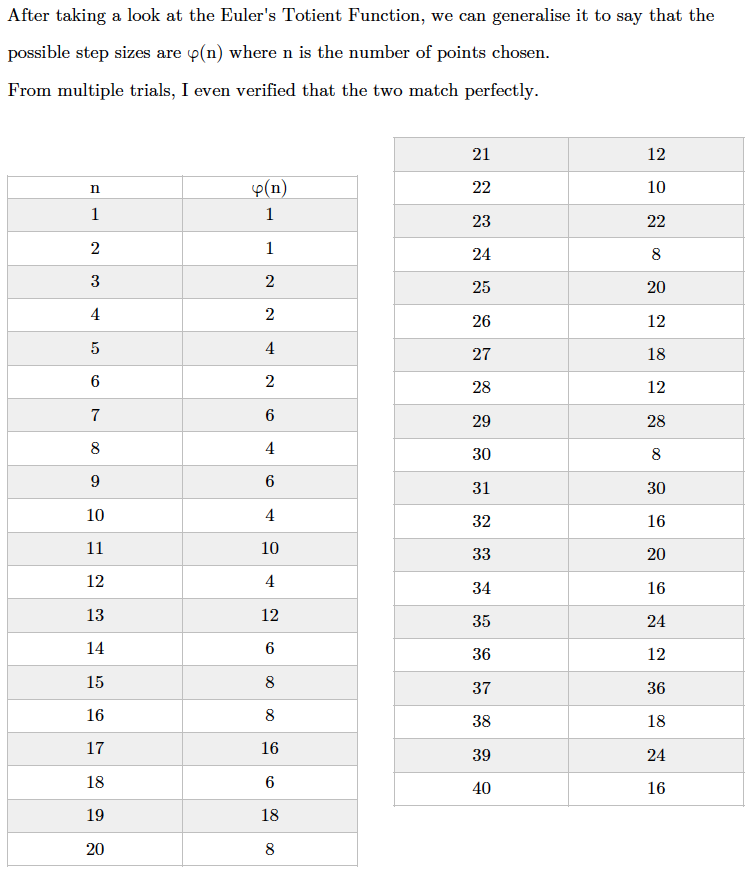#### You may also like### Gaxinta

A number N is divisible by 10, 90, 98 and 882 but it is NOT divisible by 50 or 270 or 686 or 1764. It is also known that N is a factor of 9261000. What is N?### Thirty Six Exactly

The number 12 = 2^2 × 3 has 6 factors. What is the smallest natural number with exactly 36 factors?### Strange Numbers

All strange numbers are prime. Every one digit prime number is strange and a number of two or more digits is strange if and only if so are the two numbers obtained from it by omitting either its first or its last digit. Find all strange numbers.

# Stars

##### Age 11 to 14Challenge Level

Explore what happens with different numbers of points and different step sizes. How can you work out what step sizes will visit all the points for any given number of points?

Paul from Test Valley Secondary School wrote:

If you set the Points to 8 and make the step size 3 it will hit all points.

The [angles in the] points between the edges of the stars are exactly 45 degrees. So 45 degrees $\times$ 8 (the number points) is 360 degrees, the angle of a circle. It will work no matter how many points you have.

Joshua from Davenies in England and Cameron, Jes and Vihan from ISZN in Switzerland thought about odd and even numbers of dots. Joshua wrote:

If the number of dots around the edge is odd then the number of steps can be odd or even. If the number of dots around the edge is even then the number of steps can only be odd otherwise it will go in an infinite loop in the same shape and on the same dots.

Cameron, Jes and Vihan continued:

This is because with jumps of two, half of the dots will be missed in the first round and if the number of jumps is a factor of the number of dots, then it will look like it only goes around once.

We also saw a pattern appear in the shape left between lines. We noticed that however many dots there were, the shape in the middle would be a shape with that number of sides. This is true if the lines are created with any number of jumps (except that number itself which creates no lines).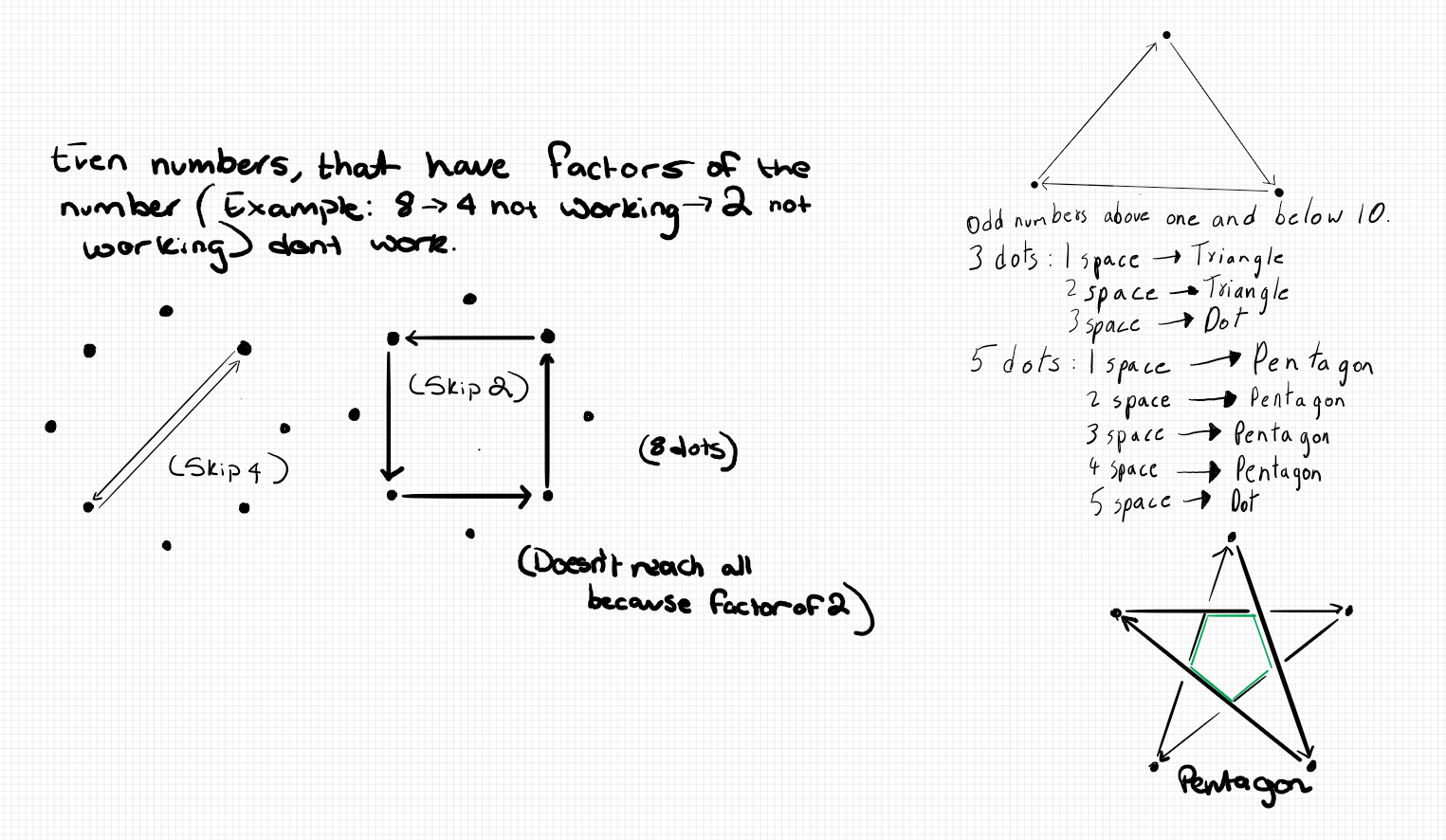Matthew from Primrose Lane Primary School noticed that:

One hits all numbers because if you move one it is bound to connect with every dot.

Pippa and Sophie from The Mount School York in the UK tried out different numbers of dots. Here is some of their work:

Circle of 8 dots
You can move around in steps of 1, 3, 5, 7

Circle of 9 dots
You can move around in steps of 1, 2, 4, 5, 7, 8

Circle of 10 dots
You can move around in steps of 1, 3, 7, 9

Click here to see a table containing Pippa and Sophie's work for 3 to 24 points.

Harvey from St Nicholas in the UK described a method for finding which step sizes will not visit all of the points:

To find it out, if the number of points is divisible by the amount you will skip, then you will not visit all the points. If we take 9 for example, and skip 3, then you will have 3 lines because 3$\times$3 = 9.

Mahdi from Mahatma Gandhi International School in India added:

My initial observations was that when you choose a step size that isn’t a factor of the number of points chosen, then we might achieve the result of striking all points. But soon, with multiple trials, I found out that for example, even though 4 is not a factor of 10, all points are not visited!? Something was off, but I couldn’t figure out why...

GG and Avirat from Wilson's Grammar School in the UK, Ivan, Jasmine and Lavender from Harrow International School HK in Hong Kong, Matthew, Pippa and Sophie and Mahdi described which step sizes will not visit all of the points. Matthew wrote:

Any factor of the number of dots fails to connect with all the dots. Also any multiple of the factor fails to hit.

Pat from Rugby School Thailand used algebraic notation and language to say the same thing (GCD = greatest common divisor = highest common factor = HCF):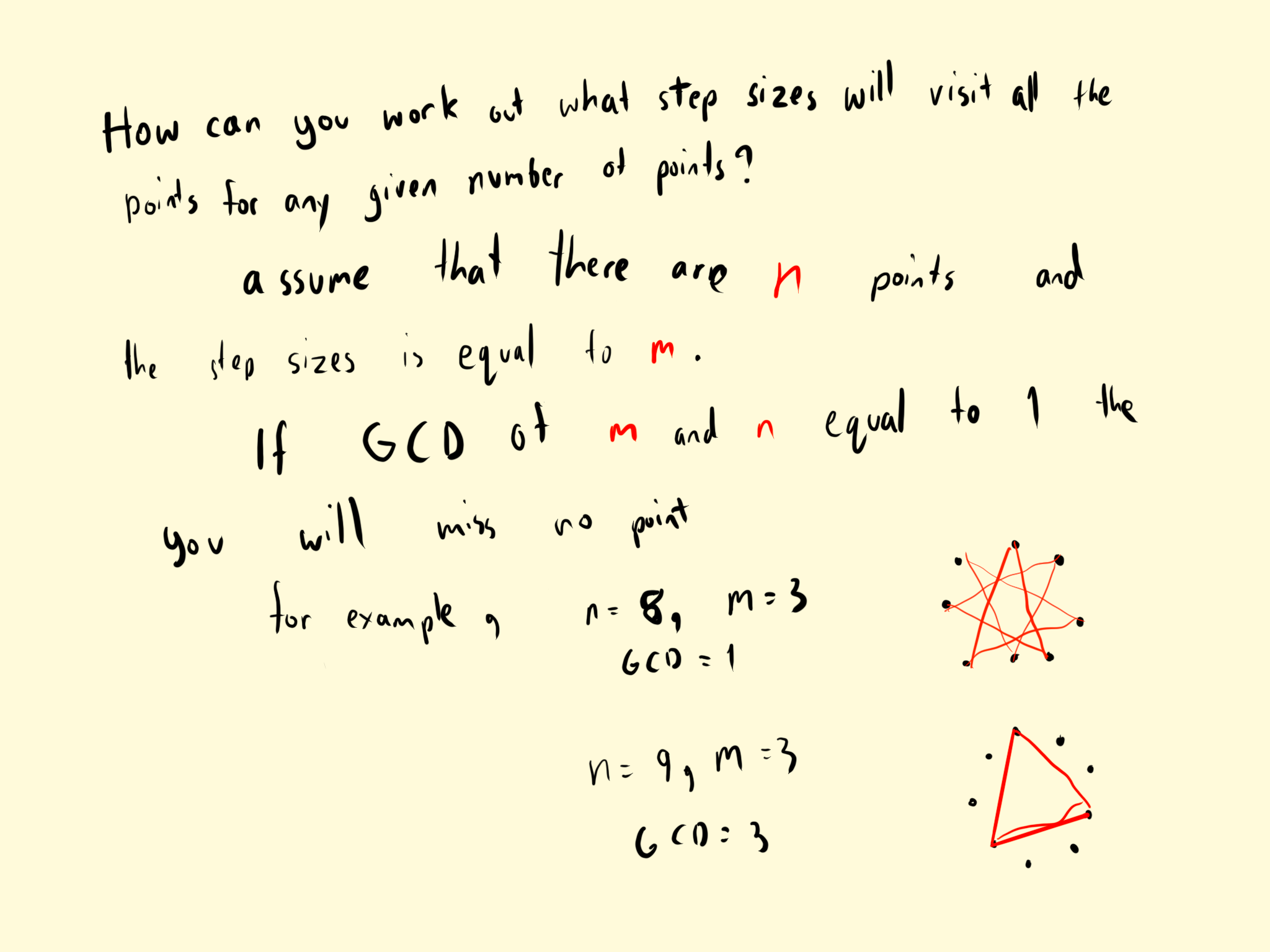Han Chin from Hong Kong and Mahdi explained why this happens. This is Han Chin's work (Han Chin says two numbers are relatively prime if their GCD is 1):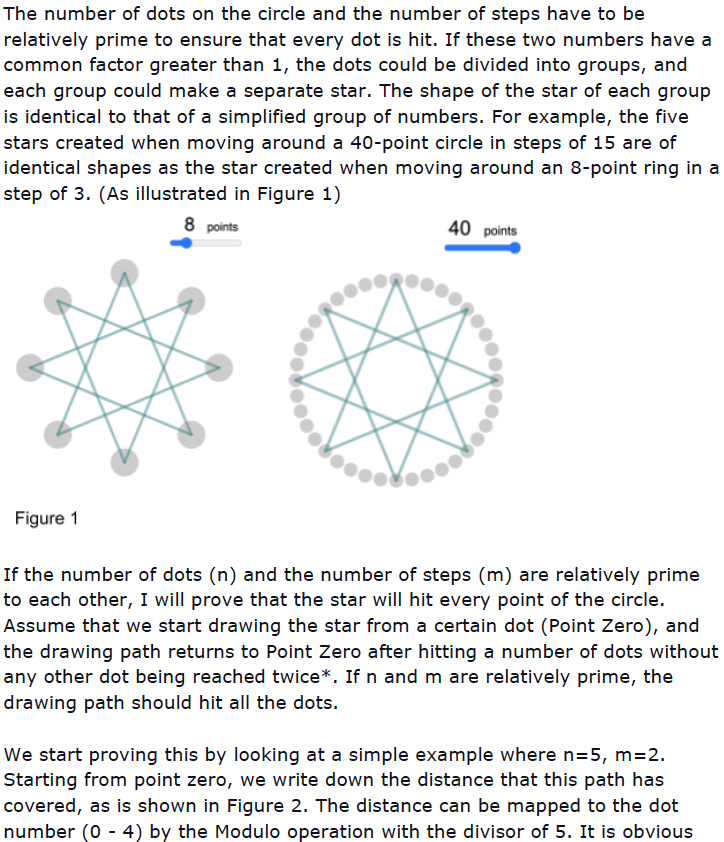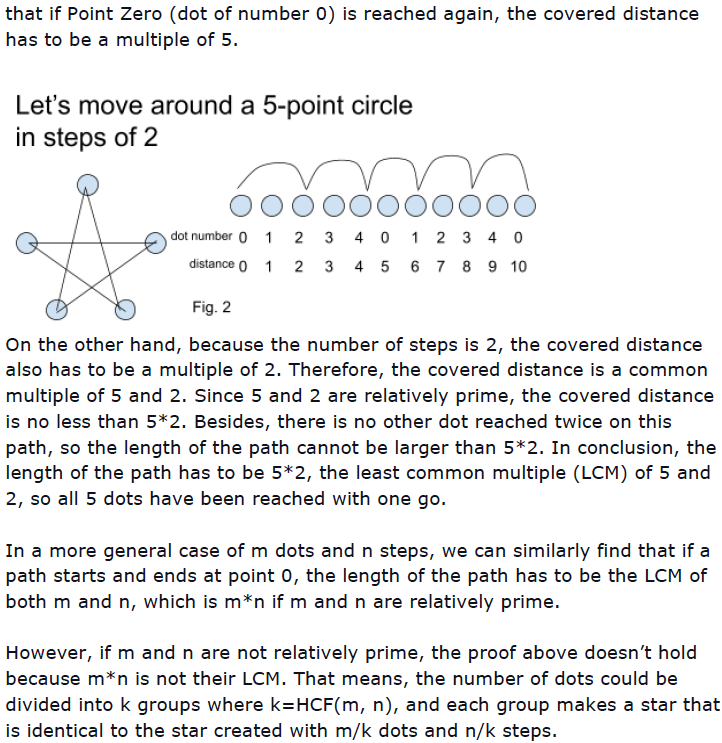Now consider $5$ points. You can visit all the points irrespective of the step size. Which other numbers have this property?

Bethan from Temple Newsom Halton Primary School wrote:

I have found that if the number of points in this problem is a prime number then all of the steps work and all of the steps join each other together whereas if the number of points is not a prime number then not all of the step [sizes] work and not all of them join each other together. I have proved this by testing my theory on different numbers of points that are prime and not prime. For example 23 is a prime number and all of its steps work whereas 21 is not a prime number and therefore not all of the steps on it work. We have also tried this with 3 points 11 points and 13 points. My theory works on all of these.

Pippa and Sophie, Matthew, Harvey and Han Chin linked this to their ideas about factors and relatively prime numbers. Matthew wrote:

Any time you have a prime number of dots, all the dots will be connected because it has no factors to miss.

Han Chin wrote that [this] is because a prime number is relatively prime to all the numbers smaller than it.

Can you find a relationship between the number of dots on the circle and the number of step sizes that will ensure that all points are hit?

Pippa and Sophie recorded the number of step sizes that hit all the points for 3 to 24 points and made some observations: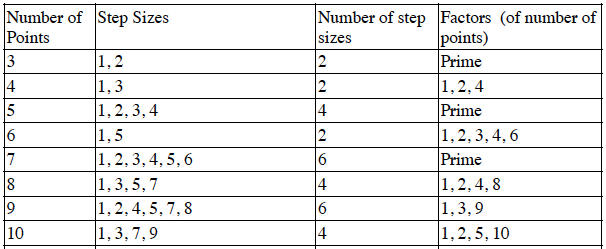If the number of points is prime the formula for the number of step sizes is $n-1$ where $n$ is the number of points.